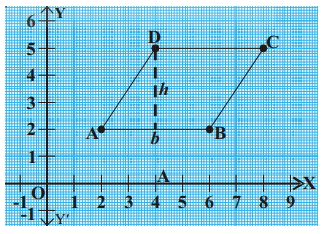# AP Board Class 9 Maths Chapter 5 Coordinate Geometry

Andhra Pradesh board Class 9 Maths Chapter 5, deals with the topic of Coordinate Geometry. In this chapter, students will learn about topics like the cartesian system and understand terms associated with the coordinate plane. The chapter also talks about other topics like;

• Locating a Point
• Origin and Coordinates of Origin.
• Plotting points in the plane and more.

## What is Coordinate Geometry?

The study of Geometry that is based on the coordinate system is known as Coordinate Geometry. Here, the position of the points on the plane is defined in terms of the ordered pair of numbers, which are the coordinates. Besides, the study of coordinates has laid down the foundation for most modern fields of Geometry, including Algebraic, Discrete, Differential and Computational Geometry.

### Cartesian System

A Cartesian system is a coordinate system that is used to specify the location of a point in the plane or in three-dimensional space. The location is specified by a set of numerical coordinates or signed distances from the coordinate axis or two fixed perpendicular oriented lines.

Most of the times, the Cartesian coordinate system is used to manipulate equations for straight lines, planes and squares which are either in two or three dimensions.

### Origin

Origin is the intersecting point between X-axis and Y-axis. Origin is taken as a reference point in locating some other points in a plane.

### Locating A Point

Locate a point on the plane is usually done using a set of two numbers. Generally, writing down the location of the two numbers on the x-axis and the y-axis will give a single position on the plane.

Students can have a look at some of the chapter questions along with their solutions below;

Question 1: In which quadrant does the point (-3,4) lie?

Solution: 2nd Quadrant. Since the point is positive for the y-axis and negative in the x-axis, thus the point must lie in the 2nd quadrant.

Question 2: What are the coordinates of the point that will divide the line joining the points (3, 5) and (11, 8) externally in the ratio 5:2.

• A. (5/3 , 1/3) B. (3/49 , 1/10) C. (49/3 , 10)

Solution: Option C

Using the formula

x = (mx2 – nx1)/(m – n)

y = (my2 – ny1)/(m – n)

where m:n is 5:2 in our case.

Using these values you will get points (49/3, 10).

Question 3: Plot the given values A(2, 2), B(6, 2), C (8, 5) and D (4, 5) in a graph sheet. Join all the points to make it a parallelogram and also find the area.Solution: All the given points lie in Q1.

From the graph b = AB = 4 units.

Height h = 3 units

Area of parallelogram = base × height

= 4 × 3 = 12 unit2

Question 4: State the abscissa and ordinate of the following point and describe the position of each point

(i) P(8,8)

(ii) Q (6, 8).

Solution:(i) P (8,8)
abscissa = 8 (x – coordinate); Ordinate = 8 (y – coordinate)
The point P is at a distance of 8 units from Y-axis measured along the positive point of X-axis from the origin. As its ordinate is 8, the point is at a distance of 8 units from X-axis measured along the positive point of Y-axis from origin.(ii) Q (6, 8)
abscissa = 6 ; Ordinate = 8
The point Q is at a distance of 6 units from Y-axis measured along positive X-axis and it is at a distance of 8 units from X-axis measured along negative Y-axis.

Stay tuned to BYJU’s to know more about AP SSC Board and access various study materials, including syllabus, exam timetable and more.